# Linear algebra for game developers ~ part 2

July 3rd, 2009

Now that I've introduced vectors in Part 1, we need to look at some of the fundamental tools for working with them. The most important tools to understand are length, normalization, distance, the dot product, and the cross product. Once you wrap your mind around these concepts, and write functions to use them, you can solve most vector problems you might encounter.

### Length

If we have a ship with velocity vector V (4,3), we might also want to know how fast it is going, in order to calculate how much the screen should shake or how much fuel it should use. To do that, we need to find the length (or magnitude) of vector V. The length of a vector is often written using || for short, so the length of V is |V|.

We can think of V as a right triangle with sides 4 and 3, and use the Pythagorean theorem to find the hypotenuse: x2 + y2 = h2. That is, the length of a vector H with components (x,y) is sqrt(x2+y2). So, to calculate the speed of our ship, we just use:

|V| = sqrt(42+32) = sqrt(25) = 5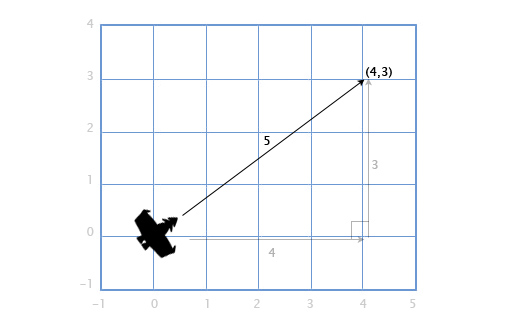This works with 3D vectors as well -- the length of a vector with components (x,y,z) is sqrt(x2+y2+z2).

### Distance

If the player P is at (3,3) and there is an explosion E at (1,2), we need to find the distance between them to see how much damage the player takes. This is easy to find by combining two tools we have already gone over: subtraction and length. We subtract P-E to get the vector between them, and then find the length of this vector to get the distance between them. The order doesn't matter here, |E-P| will give us the same result.

Distance = |P-E| = |(3,3)-(1,2)| = |(2,1)| = sqrt(22+12) = sqrt(5) = 2.23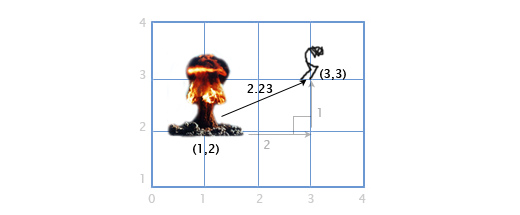### Normalization

When we are dealing with directions (as opposed to positions or velocities), it is important that they have unit length (length of 1). This makes life a lot easier for us. For example, let's say there is a gun pointing in the direction of (1,0) that shoots a bullet at 20 m/s. What is the velocity of the bullet? Since the direction has length 1, we can just multiply the direction and the bullet speed to get the bullet velocity: (20,0). If the direction vector had any other length, we couldn't do this -- the bullet would be too fast or too slow.

A vector with a length of 1 is called "normalized". So how do we normalize a vector (set its length to 1)? Easy, we divide each component by the vector's length. If we want to normalize vector V with components (3,4), we just divide each component by its length, 5, to get (3/5, 4/5). Now we can use the pythagorean theorem to prove that it has length 1:

(3/5)2 + (4/5)2 = 9/25 + 16/25 = 25/25 = 1

### Dot product

What is the dot product (written •)? Let's hold off on that for a second, and look at how we calculate it. To get the dot product of two vectors, we multiply the components, and then add them together.

(a1,a2)•(b1,b2) = a1b1 + a2b2

For example, (3,2)•(1,4) = 3*1 + 2*4 = 11. This seems kind of useless at first, but lets look at a few examples: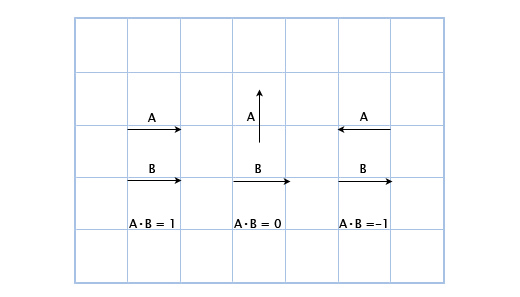Here, we can see that when the vectors are pointing the same direction, the dot product is positive. When they are perpendicular, the dot product is zero, and when they point in opposite directions, it is negative. Basically, it is proportional to how much the vectors are pointing in the same direction. This is a small taste of the power of the dot product, and it's already pretty useful!

Let's say we have a guard at position G (1,3) facing in the direction D (1,1), with a 180 field of view. We have a hero sneaking by at position H (3,2). Is he in the guard's field of view? We can find out by checking the sign of the dotproduct of D and V (the vector from the guard to the hero). This gives us:

V = H-G = (3,2)-(1,3) = (3-1,2-3) = (2,-1)
D•V = (1,1)•(2,-1) = 1*2+1*-1 = 2-1 = 1

Since 1 is positive, the hero is in the guard's field of view!We know that the dot product is related to the extent to which the vectors are pointing in the same direction, but what is the exact relation? It turns out that the exact equation for the dot product is:

AB = |A||B|cosθ

Where θ (pronounced "theta") is the angle between A and B. This allows us to solve for θ if we want to find out the angle:

θ = acos([AB]/[|A||B|]).

As I mentioned before, normalizing vectors makes our life easier! If A and B are normalized, then the equation is simply:

θ = acos(AB)

Let's revisit the guard scenario above, except the guard's field of view is only 120. First, we get the normalized vectors for the direction the guard is facing (D'), and the direction from the guard to the hero (V'). Then, we check the angle between them. If it is greater than 60 (half of the field of view), then the hero is not seen.

D' = D/|D| = (1,1)/sqrt(12+12) = (1,1)/sqrt(2) = (0.71,0.71)
V' = V/|V| = (2,-1)/sqrt(22+(-1)2) = (2,-1)/sqrt(5) = (0.89,-0.45)

θ = acos(D'V') = acos(0.71*0.89 + 0.71*(-0.45)) = acos(0.31) = 72

The angle between the center of the guard's vision and the hero is 72, so the guard does not see him!I know this looks like a lot of work, and it is, because I'm doing it by hand. However, in a program, this is pretty simple. Here is what this would like in Overgrowth using the C++ vector libraries I wrote (inspired by GLSL syntax).

```//Initialize starting vectors vec2 guard_pos = vec2(1,3); vec2 guard_facing = vec2(1,1); vec2 hero_pos = vec2(3,2);```

``` //Prepare normalized vectors vec2 guard_facing_n = normalize(guard_facing); vec2 guard_to_hero = normalize(hero_pos - guard_pos); ```

```//Check angle float angle = acos(dot(guard_facing_n, guard_to_hero));```

### Cross Product

Let's say you have a boat that has cannons that fire to the left and right. Given that the boat is facing along the direction vector (2,1), in which directions do the cannons fire? This is easy in 2D: to rotate 90 degrees clockwise, just flip the two vector components, and then switch the sign of the second component. (a,b) becomes (b,-a). So, if the boat is facing along (2,1), the right-facing cannons fire towards (1,-2). The left-facing cannons fire in the opposite direction, so we flip both signs to get: (-1,2).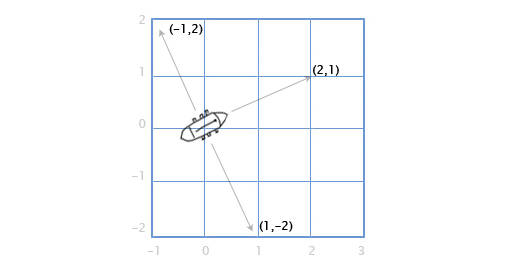So, what if we want to do this in 3D? Let's revisit our sailing ship. We have a vector for the direction of the mast M, going straight up (0,1,0), and the direction of the north-north-east wind W (1,0,2), and we want to find the direction the sail S should stick out in order to best catch the wind. The sail has to be perpendicular to the mast, and also perpendicular to the wind. To solve this, we can use the cross product: S = M x W.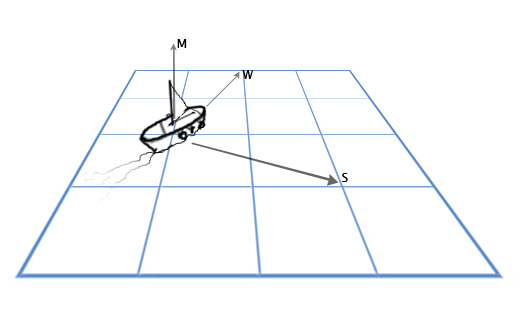The cross product of A(a1,a2,a3)) and B(b1,b2,b3)) is:

(a2b3-a3b2, a3b1-a1b3, a1b2-a2b1)

So now we can plug in our numbers and solve our problem:

S = MxW = (0,1,0)x(1,0,2) = ([1*2-0*0], [0*1-0*2], [0*0-1*1]) = (2,0,-1)

This is pretty ugly to do by hand. For most graphics and game work I would recommend just encapsulating it in a function like the one below, and never thinking about the details again.

```vec3 cross(vec3 a, vec3 b) {     vec3 result;     result = a * b - a * b;     result = a * b - a * b;     result = a * b - a * b;     return result; }```

Another common use for the cross product in games is to find surface normals -- the direction that a surface is facing. For example, let's take a triangle with vertex vectors A, B and C. How do we find the direction that the triangle is facing? It seems tricky, but we have the tools to do it now. We can use subtraction to get the direction from A to C (C-A) 'Edge 1' and A to B (B-A) 'Edge 2', and then use the cross product to find a new vector N perpendicular to both of them... the surface normal.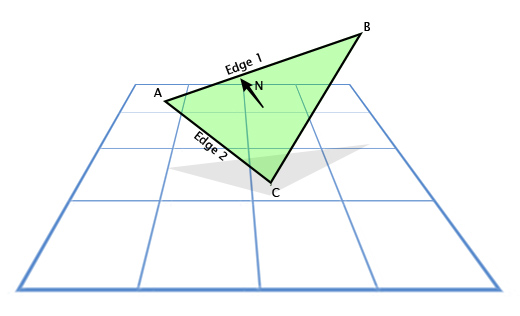Here is what that would like in code:

```vec3 GetTriangleNormal(vec3 a, vec3 b, vec3 c) {     vec3 edge1 = b-a;     vec3 edge2 = c-a;     vec3 normal = cross(edge1,edge2);     return normal; }```

Fun fact: the basic expression for lighting in games is just N•L, where N is the surface normal, and L is the normalized light direction. This is what makes surfaces bright when they face towards the light, and dark when they don't.

### Next time

We covered what vectors are in Part 1, and now we have a solid toolbox for working with them. Next, I would like to talk about vector spaces and matrix transformations.

This post was a lot more complicated than the last one -- is there anything confusing here that you have questions about?